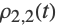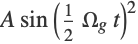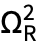# Two-Level System

A two-level atomic system subject to an optical field is analyzed.

## Time-Dependent Solution and the Rabi Frequency

We define an atomic system consisting of two states (a ground state labeled 1 and upper state labeled 2). This is a "toy" system that neglects angular momentum (J and M are not defined). We apply a light field detuned from resonance by a frequency Δ.

We first neglect relaxation and solve for the dynamics of the system.

 Define the atomic system.
 Here is what the density matrix looks like.
 Define the optical field with frequency ω. This is an x-polarized field by default, but for a toy system the polarization does not matter.
 The Hamiltonian for the system subject to the optical field.
 For simplicity of notation, we define the Rabi frequency ΩR=(1||d1||2) E0.
 Apply the rotating-wave approximation to the Hamiltonian. We define the detuning Δ=ω-Energy.
 Here are the evolution equations.
 Here are initial conditions, putting all the population in the ground state at t=0.

Here is the solution.

 Solve the system of equations with DSolve. We see that the upper state has populationgiven bywith generalized Rabi frequencyand amplitude A=ΩR2/(ΩR2+Δ2).
 Here is a plot of the populations.

## Steady-State Solution and the Saturation Parameter

If we add relaxation to the system, we can find a steady-state solution.

 First we remove the time dependence from the density-matrix-element notation. This will have the effect of setting time derivatives of the density-matrix elements to zero.
 The density matrix with no explicit time dependence.

There are two forms of relaxation present: intrinsic relaxation of the upper state due to spontaneous decay and "transit" relaxation due to atoms leaving the system. A "relaxation matrix" accounts for these processes.

 IntrinsicRelaxation and TransitRelaxation supply the relaxation matrices.

The ground state is repopulated by the same two mechanisms: spontaneous decay transfers atoms from the upper state to the ground state, and ground-state atoms enter the system. This is taken into account by the "repopulation matrix":

 OpticalRepopulation and TransitRepopulation supply the repopulation matrices.

Here are the evolution equations, including relaxation and repopulation. Since the density matrix elements are now explicitly time independent, we obtain time-independent equations for the steady state.

 Create evolution equations with LiouvilleEquation.

Here are the solutions.

We can simplify these solutions by assuming that the transit rate is much slower than the natural width of the upper state, and by writing them in terms of the upper-state saturation parameter κ=/Γ2.

 Set γ equal to zero and substitute for ΩR.

We see that the upper-state population is a power-broadened Lorentzian in detuning. Here is a plot of the upper-state population as a function of detuning for various values of the saturation parameter.

Setting the detuning to zero, we see why κ is called the saturation parameter for this system: when κ is small, the upper-state population is linear in κ; when κ is increased to unity, the upper-state population begins to saturate, and approaches its limiting value of 1/2.